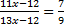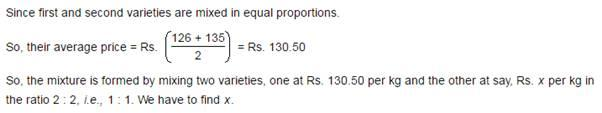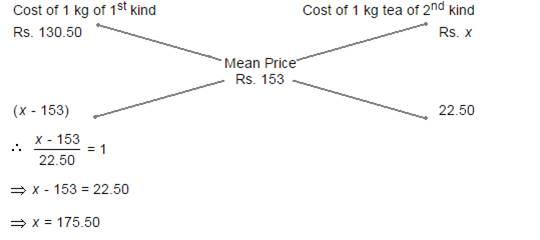## Ratio and Proportion For SBI PO : Set – 07

1) The railway fares of air conditioned sleeper and ordinary sleeper class are in the ratio 4:1. The number of passengers travelled by air conditioned sleeper and ordinary sleeper classes were in the ratio 3:25. If the total collection was Rs. 37,000, how much did air conditioner sleeper passengers pay?

a) Rs. 15,000

b) Rs. 10,000

c) Rs. 12,000

d) Rs. 16,000

e) None of these

c)

Railway fares of AC: Sleeper = 4 : 1

AC passengers : Sleeper passengers = 3:25

Hence for calculating total calculation, (4*3)+(1*25)

12x + 25x = 37000

37x = 37000

x = 1000

Cost of AC passenger pay = 3 × 4 × 1000

=Rs.12000

2) If the ratio between the profit and sale price of an article is 1:5, then the ratio between the sale price and the cost price of that article is

a) 3:2

b) 4:3

c) 5:4

d) 6:5

e) None of these

c)

Profit : S.P = 1:5

C.P = 5 – 1 = 4

S.P : C.P = 5:4

3) An office opens at 10 AM and closes at 5 PM. The lunch interval is 30 minutes. The ratio of lunch interval to the total period of office hours is

a) 1:7

b) 1:14

c) 7:1

d) 14:1

e) None of these

b)

10 am to 5 pm = 7 hours

Lunch time = 30 minutes = 1/2 hrs.

Ratio = 1/2:7

Lunch period : Total period = 1:14

4) Between two consecutive years my incomes are in the ratio of 2:3 and expenses are in the ratio 5:9 If my income in the second year is Rs. 45000 and my expenses in the first year is  Rs. 25000, my total saving for the two years is

a) 0

b) Rs. 15000

c) Rs. 20000

d) Cannot be determined

e) None of these

d)

Income in 2nd year Rs.45,000

Income in 1st year = Rs.10,000

Expense in 2nd year = Rs.25,000

Expense in 2nd year = Rs.45,000

So total savings = 75,000-7000=5000

Rs.5000

5) If the digits of the age of Mr. Ram are reversed then the new age so obtained is the age of his brother. 1/11 of the sum of their ages is equal to the difference between their ages. If Mr. Ram is elder than his brother  then find ratio of Ram and his brother ages.

a) 5:4

b) 4:5

c) 7: 6

d) 6: 5

e)  None of these

d)

Ram’s age =xy = 10x+y

Brother’s age = yx = 10y + x

1/11(10x+y+10y+x)=10x+y-10y-x

1/11(11x+11y)=9x-9y

x+y=9x-9y

10y=8x

y/x=4/5

Ram’s age =54; Brother’s age=45

Ratio = 54:45=6:5

6) The ratio of the present age of Manoj to that of Wasim is 3: 11. Wasim is 12 year younger than Rehana, Rehana’s age after 7 year will be 85 year. Waht is the present age of Manoj’s father, who is 25 year older Manoj?

a) 43 year

b) 67 year

c) 45 year

d) 69 year

e) None of these

a)

According to question,

Let assume, Manoj =M, Wasim = W, Rehana = R

Manoj’s father = MF

The present age of Monaj and Wasim are 3x, 11x

If, W = (R – 12); R + 7 = 85; Mf = 25 + M

W = R -12 => R + 7 = 85 => R = 78

So, W = 78 – 12 = 66

11x = 66 => x = 6

The present age of Manoj = 3*6=18

MF=25+18=43

= 43 years

7) A certain amount was to be distributed among A, B and C in the ratio 2 : 3 : 4, but was erroneously distributed in the ratio 7 : 2 : 5. As a result of this, B received Rs. 40 less. What is the actual amount?

a) Rs. 210

b) Rs. 270

c) Rs. 230

d) Rs. 280

e) None of these

a)

B’s Share =3x/9

Due to error B’s Share = 2x/14

Difference in B’s share due to error = 40

3x/9-2x/9=40

42x-18x/126=40

24x=40*126

x= Rs.210

8) Two numbers are in the ratio 11:13. If 12 be subtracted from each, the remainders are in the ratio 7:9.Find out the numbers.

a) 22 and 26

b) 33 and 39

c) 44 and 52

d) Cannot be determined

e) None of these

b)

11:1399 – 108 = 91 – 84

8 = – 84 + 108

8 = 24

= 3

9) A rectangular field has its length and breadth in the ratio 6:5 respectively. A man riding a bicycle completes one loop of this field along its perimeter at the speed of 19.8 km/hr in 2 minute. What is the area of the field ?

a) 19200 m2

b) 27000 m2

c) 19300 m2

d) 32500 m2

e)

None of these

b) D= S × t = 19.8 × 2/60= 0.66 km= 660m

L= 6/11*660/2= 180m; b=5/11*660/2 = 150m

Area= 180 × 150= 27000 m2

10) Tea worth Rs. 126 per kg and Rs. 135 per kg are mixed with a third variety of tea in the ratio 1 : 1 : 2. If the mixture is worth Rs. 153 per kg, what is the price of the third variety per kg?

a) Rs.182.50

b) Rs.170.5

c) Rs.175.50

d) Rs.180

e) None of these